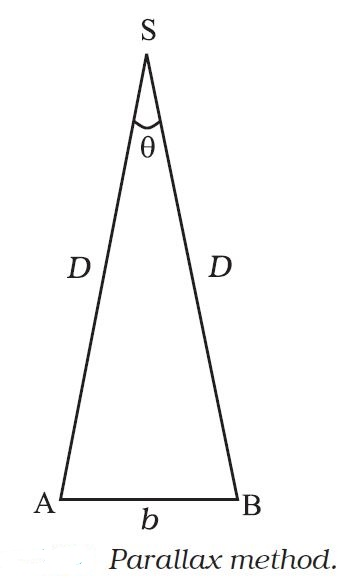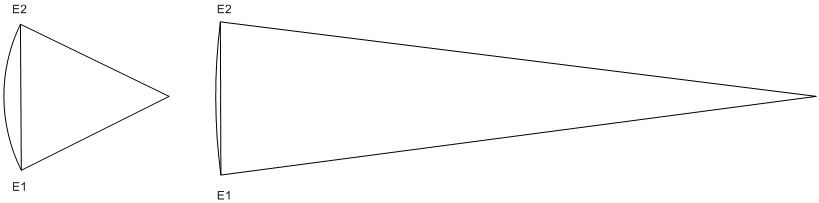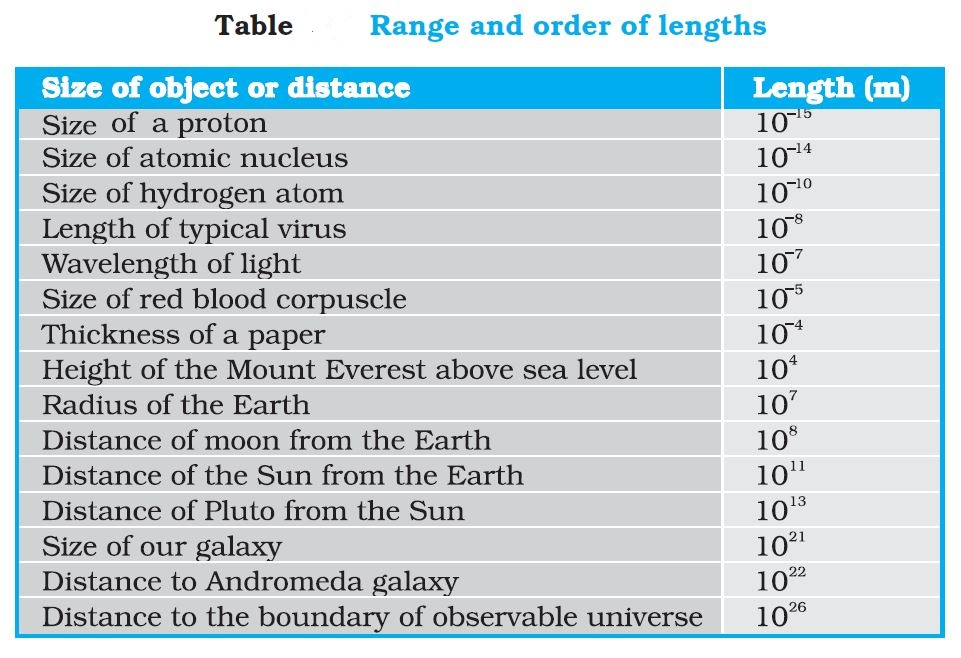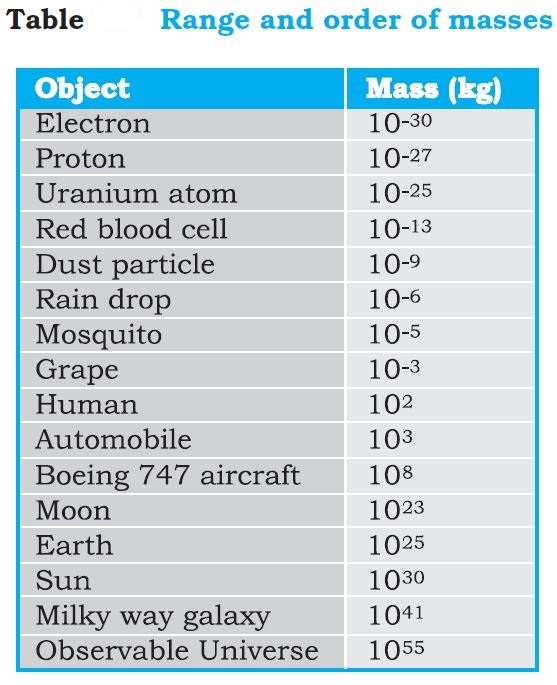Physics MEASUREMENT OF LENGTH, MASS AND TIME
Click for Only Video

### Topics Covered• Measurement of Large Distances
• Parallax Method
• Parallax
• Basis
• Parallax or Parallactic Angle
• Estimation of Very Small Distances: Size of a Molecule
• Range of Lengths
• Measurement of Mass
• Unified Atomic Mass Unit
• Range of Masses
• Measurement of Time
• Atomic Clock

### Measurement of Length

Some familiar direct methods for the measurement of length - meter scale (10^(-3)m to 10^2 m), vernier calliper (accuracy of 10^(-4)m), screw gauge and spherometer (upto 10^(-5)m).

To measure lengths beyond these ranges, we make use of some special indirect methods.

### Measurement of Large DistancesLarge distances such as the distance of a planet or a star from the earth cannot be measured directly with a metre scale. An important method in such cases is the parallax method.

text(Parallex)
Parallax is a displacement or difference in the apparent position of an object viewed along two different lines of sight, and is measured by the angle or semi-angle of inclination between those two lines.

text(Basis)
The distance between the two points of observation is called the basis.

text(Example-) When you hold a pencil in front of you against some specific point on the background (a wall) and look at the pencil first through your left eye A (closing the right eye) and then look at the pencil through your right eye B (closing the left eye), you would notice that the position of the pencil seems to change with respect to the point on the wall. This is called parallax. In this example, the basis is the distance between the eyes.

text(To measure the distance D of a far away planet S by the parallax method)
We observe it from two different positions (observatories) A and B on the Earth, AB=b
The ∠ASB in Fig. represented by symbol θ is called the text(parallax angle) or text(parallactic angle).
As the planet is very far away, b/D > > 1 :. theta is very small.
Then we approximately take AB=b= arc of a circle with centre at S
D=AS=BS= radius
=> AB=b=D theta where θ is in radians.
D=b/thetatext(Size or Angular Diameter of Planet)
If d= diameter of the planet
alpha= angular size of the planet (the angle subtended by d at the earth)
we have, alpha=d/D

### Estimation of Very Small Distances: Size of a Molecule

A simple method for estimating the molecular size of oleic acid is given below. Oleic acid is a soapy liquid with large molecular size of the order of 10^(–9) m.

• The idea is to first form mono-molecular layer of oleic acid on water surface.

We dissolve 1 cm^3 of oleic acid in alcohol to make a solution of 20 cm^3. Then we take 1 cm^3 of this solution and dilute it to 20 cm^3, using alcohol.
So, the concentration of the solution is equal to (1/(20xx20))cm^3 of oleic acid/cm^3 of solution.

Next we lightly sprinkle some lycopodium powder on the surface of water in a large trough and we put one drop of this solution in the water. The oleic acid drop spreads into a thin, large and roughly circular film of molecular thickness on water surface. Then, we quickly measure the diameter of the thin film to get its area A.

Suppose we have dropped n drops in the water.
Volume of n drops of solution =nV cm^3
Amount of oleic acid in this solution =nV(1/(20xx20))cm^3
This solution of oleic acid forms a very thin layer of thickness t, then the thickness of the film
t=text(Volume of the film)/text(Area of the film)
t=(nV)/(20xx20) cm
If we assume that the film has mono-molecular thickness, then this becomes the size or diameter of a molecule of oleic acid.

The value of this thickness comes out to be of the order of 10^(–9) m.

### Range of Lengths• The sizes of the objects may vary from the size of the order of 10^(–14) m of the tiny nucleus of an atom to the size of the order of 10^(26) m of the extent of the observable universe.

• Table gives the range and order of lengths and sizes of some of these objects.

• Some special length units for short and large lengths
1 text(fermi) = 1 f = 10^(-15) m
1 text(angstrom) = 1 Å = 10^(–10) m
1 text(astronomical unit) = 1 AU (average distance of the Sun from the Earth) = 1.496 × 10^(11) m
1 text(light year) = 1 ly= 9.46 × 10^(15) m (distance that light travels in 1 year)
1 text(parsec) = 3.08 × 10^(16) m (Parsec is the distance at which average radius of earth’s orbit subtends an angle of 1 arc second)

### Measurement of Mass

• Mass is a basic property of matter. It does not depend on the temperature, pressure or location of the object in space.
• SI unit of mass = kilogram(kg)

text(Unified Atomic Mass Unit) (u)
While dealing with atoms and molecules, the kilogram is an inconvenient unit. In this case, there is an important standard unit of mass, called the unified atomic mass unit.

1 unified atomic mass unit = 1 u
= (1/12) of the mass of an atom of carbon-12 isotope (text()_6^12 C) including the mass of electrons
=1.66xx10^(-27)kg

• Mass of commonly available objects can be determined by a common balance like the one used in a grocery shop.
• Large masses in the universe like planets, stars, etc., based on Newton’s law of gravitation can be measured by using gravitational method.
• For measurement of small masses of atomic/subatomic particles etc., we make use of mass spectrograph in which radius of the trajectory is proportional to the mass of a charged particle moving in uniform electric and magnetic field.

### Range of MassesThe masses of the objects may vary from tiny mass of the order of 10^(-30) kg of an electron to the huge mass of about 10^(55) kg of the known universe.

Table gives the range and order of the typical masses of various objects.

### Measurement of TimeAny phenomenon that repeats itself regularly can be used as a time-measuring device. For example, a wall clock makes use of an oscillating pendulum, while wrist watches are based on the vibrations of mass-spring system. Of the many repetitive phenomena in nature, the axial rotation of earth determines the length of the day. One (mean solar) second has been defined to be 1/86,400 of a (mean solar) day.
Some devices used to measure very small and very large time-intervals are as follows :
text(Atomic Clocks)
• One of the most accurate atomic clocks is the cesium clock. It is based on a characteristic time-period of the microwave radiation emitted by cesium-133 atom. Two modern cesium clocks could run for 300,000 years before their readings would differ by more than 1 s. Atomic clocks are used to measure time-intervals of the order of 10^(-12) s.
• In the cesium atomic clock, the second is taken as the time needed for 9,192,631,770 vibrations of the radiation corresponding to the transition between the two hyperfine levels of the ground state.

text(Quartz-crystel Clocks)
These clocks are based on the electrically-sustained vibrations of a quartz crystal. These vibrations are produced by applying an oscillating emf across a pair of faces of the crystal. The quartz clock can be calibrated against the rotating earth by astronomical observations. The best quartz clock has shown a maximum error of 5mu s in 1 year.

text(Decay of Elementary Particles)
The life-times of many unstable elementary particles range from 10^(-16) s to 10^(-20)s. Time-intervals of these orders can be measured by studying photographically the decay of these particles.

text(Radioactive Dating)
The decay of very long-lived radioactive elements have led to estimate the ages of fossils, old rocks and earth. If a radioactive element and its decay products remain trapped in a rock (since it first solidified), then the measurement of the proportion of the end-product to the parent element gives the age of the rock.
Carbon dating has been used to. estimate the age of fossils, while uranium dating to estimate the age of earth which is about 4 xx 10^9 years.